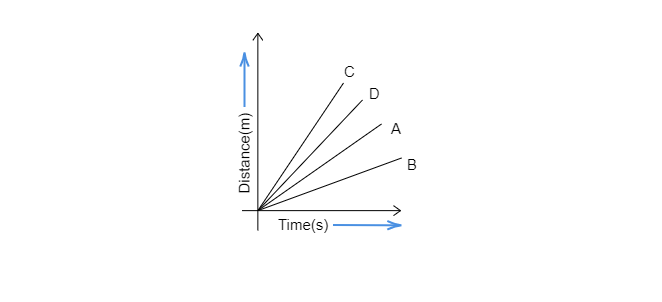$(a)$. car A is faster than car D.
$(b)$. car B is the slowest
$(c)$. car D is faster than the car C
$(d)$. car C is the slowest"
">

# Four cars A, B, C and D are moving on a levelled, straight road. Their distance-time graphs are shown in the given figure. Which of the following is the correct statement regarding the motion of these cars?$(a)$. car A is faster than car D.$(b)$. car B is the slowest$(c)$. car D is faster than the car C$(d)$. car C is the slowest"

From the given graph we can conclude that car B is the slowest.

So, option (b) is correct.

Updated on: 10-Oct-2022

23 Views